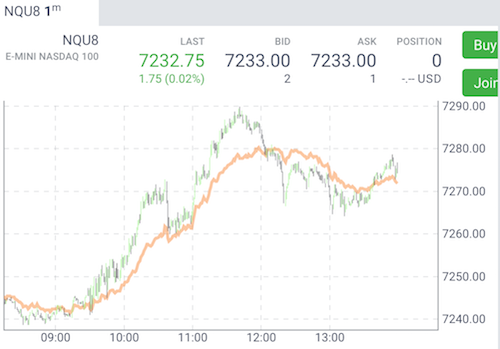Options
All
• Public
• Public/Protected
• All

Fourier Moving Average

Lodash is a very popular and high-performance library to work with arrays and objects. All you need to do is to include const lodash = require("lodash") in your module.

Another is a library for Fast Fourier Transform. Here is an example of how to apply this library to build a moving average that calculated as FFT of input data, a filter of high frequencies, and inverse FFT.

const predef = require("./tools/predef");
const FFT = require("fft");

class fourierMA {
init() {
const period = this.props.period;
this.fft = FFT(period);
this.signal = new Array(period);
this.zero = new Array(period);
for(let i=0; i<period; ++i) {
this.zero[i] = 0.0;
}
this.lastIndex = -1;
}

map(d, index) {
const period = this.props.period;
const value = d.value();

if (index < period) {
this.signal[period - index - 1] = value;
}
else {
if (this.lastIndex < index) {
this.signal.pop();
this.signal.unshift(value);
}
else {
this.signal = value;
}
}

this.lastIndex = index;

if (index >= period) {
const re = [].concat(this.signal);
const im = [].concat(this.zero);
this.fft.fft1d(re, im);

const middle = period / 2 + 1;
const startFreq = this.props.filterFreqStart;
for(let i=startFreq; i<middle; ++i) {
re[i] = im[i] = 0.0;
re[period - i] = im[period - i] = 0.0;
}
this.fft.ifft1d(re, im);

return re;
}
}
}

module.exports = {
name: "fourierMA",
description: "Fourier MA",
calculator: fourierMA,
params: {
period: predef.paramSpecs.period(64),
filterFreqStart: predef.paramSpecs.period(16),
},
tags: ["My Indicators"],
};Generated using TypeDoc